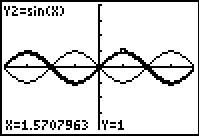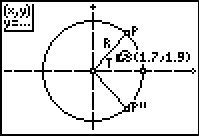••• ##### Device
• TI-83 Plus Family
• TI-84 Plus
• TI-84 Plus Silver Edition

# Algebra II: Proof of Identity

by Texas Instruments#### Overview

Students use graphs to verify the reciprocal identities. They then use the calculator's manual graph manipulation feature to discover the negative angle, cofunction, and Pythagorean trigonometric identities.

#### Key Steps

•In problem 1, students will investigate the idea that two functions are equal if their graphs are exactly the same.

Students will also investigate the reciprocal of an identity. Students should se that a reciprocal identity shows that one trigonometric function is equal to the reciprocal of another.

•In problem 2, students further explore negative angle identities.

Students see will observe two ways to translate sin(x) to make it line up with sin(–x). They will see that setting both functions to be equal to each other they will get sin(–x) = –sin(x).

•Problem 2 gives students the opportunity to explore cofunction identities. They will see that sine and cosine functions are similar to each other, as compared to the tangent function.

Students graph sin(x) and cos(–x). Students will learn that the idea of sine and cosine being similar can be formalized by the cofunction identities.